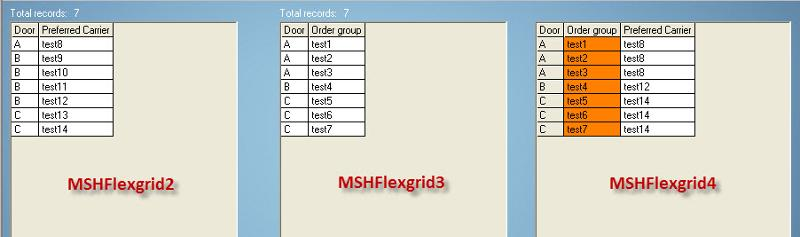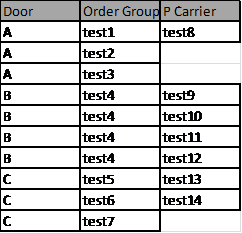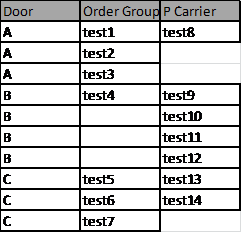# VB6 - Transfer  data from 1 grid to anotheron
Hello all

I have this code bellow that transfer data from 1 grid to another.

The issue i have is with this part of the code:
``````    For z = MSHFlexGrid2.Rows - 1 To 0 Step -1
For zz = 1 To MSHFlexGrid2.Rows - 1
If MSHFlexGrid4.TextMatrix(z, 0) = MSHFlexGrid2.TextMatrix(zz, 0) Then

MSHFlexGrid4.TextMatrix(z, 2) = MSHFlexGrid2.TextMatrix(zz, 1)
MSHFlexGrid4.Row = z
MSHFlexGrid4.Col = 1
MSHFlexGrid4.CellBackColor = &H80FF&

End If
Next zz
Next z
``````

The Preferred carrier column from MSHFlexgrid4 should not have duplicate value. If it was transferred once, it should not show twice based on the DOOR letter.

Then, if for example, in grid 4, i only have 1 door B but in grid 2, i have 4 B door, then it should add a new row, add Door B in column 0 and add the value in column Preferred carrier column from MSHFlexgrid4.How can i do that please?

FULL CODE:
``````    Dim i As Integer

For i = 0 To MSHFlexGrid3.Rows - 1
If MSHFlexGrid3.TextMatrix(i, 0) <> "" Then
MSHFlexGrid4.TextMatrix(i, 0) = MSHFlexGrid3.TextMatrix(i, 0)
MSHFlexGrid4.TextMatrix(i, 1) = MSHFlexGrid3.TextMatrix(i, 1)
MSHFlexGrid4.Rows = MSHFlexGrid4.Rows + 1
End If
Next i

'Delete empty row
Dim j As Integer
Dim k As Integer
Dim sSQL As String
For k = MSHFlexGrid4.Rows - 1 To 1 Step -1
sSQL = ""
For j = 1 To MSHFlexGrid4.Cols - 1
sSQL = sSQL & Trim(MSHFlexGrid4.TextMatrix(k, j))
Next
If Trim(sSQL) = "" Then
MSHFlexGrid4.RemoveItem k
End If
If MSHFlexGrid4.Rows <= 1 Then Exit For
Next

Dim z As Integer
Dim zz As Integer
MSHFlexGrid4.Cols = MSHFlexGrid4.Cols + 1
MSHFlexGrid4.TextMatrix(0, 2) = "Preferred Carrier"

For z = MSHFlexGrid2.Rows - 1 To 0 Step -1
For zz = 1 To MSHFlexGrid2.Rows - 1
If MSHFlexGrid4.TextMatrix(z, 0) = MSHFlexGrid2.TextMatrix(zz, 0) Then

MSHFlexGrid4.TextMatrix(z, 2) = MSHFlexGrid2.TextMatrix(zz, 1)
MSHFlexGrid4.Row = z
MSHFlexGrid4.Col = 1
MSHFlexGrid4.CellBackColor = &H80FF&

End If
Next zz
Next z
``````
Comment
Watch Question

Do more withEXPERT OFFICE® is a registered trademark of EXPERTS EXCHANGE®
Top Expert 2016

Commented:
Hi,

If I  understand it well you trying to do thisOrnot repeating the preferred carrier but insert new lines when there are more than in order group.

Right?

Regards

Commented:
Hi again

I would say your option 2 and also yes to not repeating the preferred carrier but insert new lines when there are more than in order group.

Thank you so much for your help. :)
try this

Dim Doors As New Collection
Dim Door_Entry As String
Dim Order_Group() As String
Dim P_Carrier() As String
Dim Carrier() As String

With MSHFlexGrid1
.FixedCols = 0
.Cols = 2
.Rows = 1
.AddItem "A" & vbTab & "test1", 1
.AddItem "A" & vbTab & "test2", 2
.AddItem "A" & vbTab & "test3", 3
.AddItem "B" & vbTab & "test4", 4
.AddItem "C" & vbTab & "test5", 5
.AddItem "C" & vbTab & "test6", 6
.AddItem "C" & vbTab & "test7", 7
.FixedRows = 1
.TextMatrix(0, 0) = "Door"
.TextMatrix(0, 1) = "Order Group"
End With

With MSHFlexGrid2
.FixedCols = 0
.Cols = 2
.Rows = 1
.AddItem "A" & vbTab & "test8", 1
.AddItem "B" & vbTab & "test9", 2
.AddItem "B" & vbTab & "test10", 3
.AddItem "B" & vbTab & "test11", 4
.AddItem "B" & vbTab & "test12", 5
.AddItem "C" & vbTab & "test13", 6
.AddItem "C" & vbTab & "test14", 7
.FixedRows = 1
.TextMatrix(0, 0) = "Door"
.TextMatrix(0, 1) = "P Carrier"
End With

With MSHFlexGrid3
.FixedCols = 0
.Cols = 2
.Rows = 1
.AddItem "A" & vbTab & "test15", 1
.AddItem "A" & vbTab & "test16", 2
.AddItem "A" & vbTab & "test17", 3
.AddItem "A" & vbTab & "test18", 4
.AddItem "B" & vbTab & "test19", 5
.AddItem "C" & vbTab & "test20", 6
.AddItem "C" & vbTab & "test21", 7
.FixedRows = 1
.TextMatrix(0, 0) = "Door"
.TextMatrix(0, 1) = "Carrier"
End With

With MSHFlexGrid4
.FixedCols = 0
.Cols = 4
.FixedRows = 1
.TextMatrix(0, 0) = "Door"
.TextMatrix(0, 1) = "Order Group"
.TextMatrix(0, 2) = "P Carrier"
.TextMatrix(0, 3) = "Carrier"
End With

End Sub

Private Sub Command1_Click()
Dim Door_Found As Boolean
Dim i As Integer

'get all distinct Doors
For i = 1 To MSHFlexGrid1.Rows - 1
If MSHFlexGrid1.TextMatrix(i, 0) <> "" Then
Door_Found = False
For Each Item In Doors
If Item = MSHFlexGrid1.TextMatrix(i, 0) Then
Door_Found = True
Exit For
End If
Next

If Door_Found = False Then
End If
End If
Next i

For Each Item In Doors

'check Order Group Tests
ReDim Order_Group(0 To 0)
For i = 1 To MSHFlexGrid1.Rows - 1
If MSHFlexGrid1.TextMatrix(i, 0) <> "" Then
If Item = MSHFlexGrid1.TextMatrix(i, 0) Then
Order_Group(UBound(Order_Group())) = MSHFlexGrid1.TextMatrix(i, 1)
ReDim Preserve Order_Group(0 To UBound(Order_Group()) + 1)
End If
End If
Next i

'check P Carrier Test
ReDim P_Carrier(0 To 0)
For i = 1 To MSHFlexGrid2.Rows - 1
If MSHFlexGrid2.TextMatrix(i, 0) <> "" Then
If Item = MSHFlexGrid2.TextMatrix(i, 0) Then
P_Carrier(UBound(P_Carrier())) = MSHFlexGrid2.TextMatrix(i, 1)
ReDim Preserve P_Carrier(0 To UBound(P_Carrier()) + 1)
End If
End If
Next i

'check Carrier Test
ReDim Carrier(0 To 0)
For i = 1 To MSHFlexGrid3.Rows - 1
If MSHFlexGrid3.TextMatrix(i, 0) <> "" Then
If Item = MSHFlexGrid3.TextMatrix(i, 0) Then
Carrier(UBound(Carrier())) = MSHFlexGrid3.TextMatrix(i, 1)
ReDim Preserve Carrier(0 To UBound(Carrier()) + 1)
End If
End If
Next i

j = 0
Door_Entry = ""
Do While j < UBound(Order_Group()) Or _
j < UBound(P_Carrier()) Or _
j < UBound(Carrier())

Door_Entry = Item

If j < UBound(Order_Group()) Then
Door_Entry = Door_Entry & vbTab & Order_Group(j)
Else
Door_Entry = Door_Entry & vbTab & " "
End If

If j < UBound(P_Carrier()) Then
Door_Entry = Door_Entry & vbTab & P_Carrier(j)
Else
Door_Entry = Door_Entry & vbTab & " "
End If

If j < UBound(Carrier()) Then
Door_Entry = Door_Entry & vbTab & Carrier(j)
Else
Door_Entry = Door_Entry & vbTab & " "
End If

j = j + 1
Loop
Next

End Sub